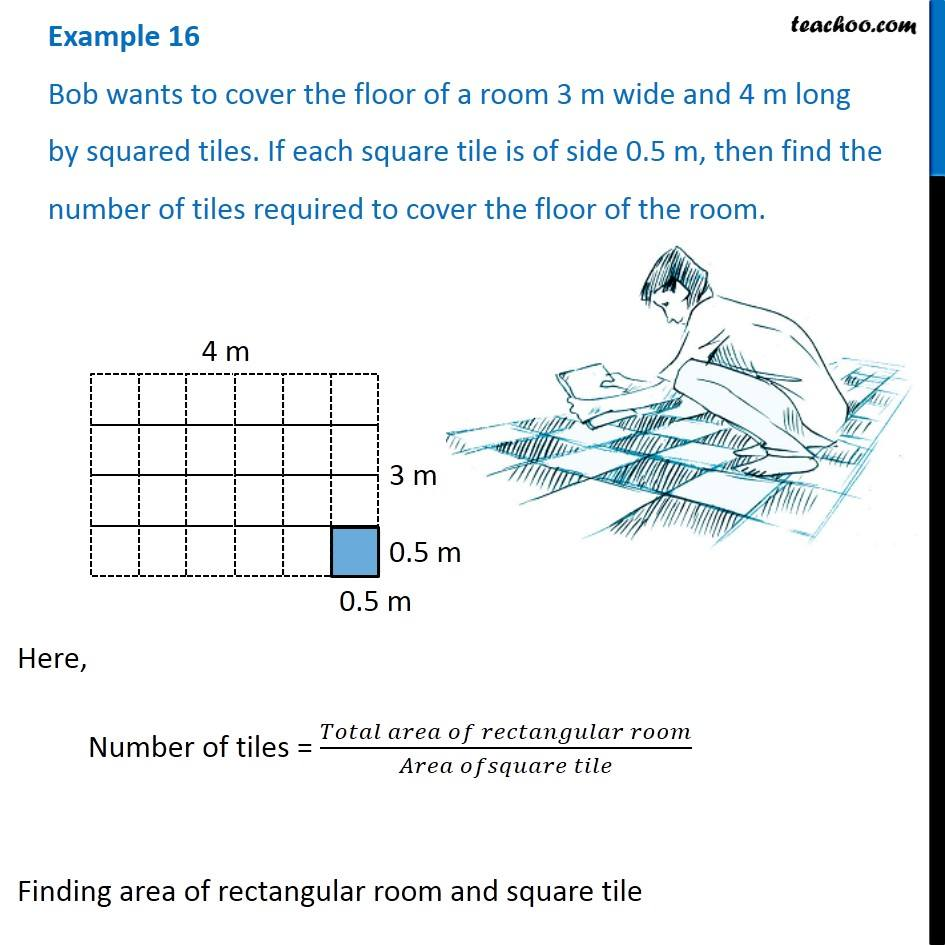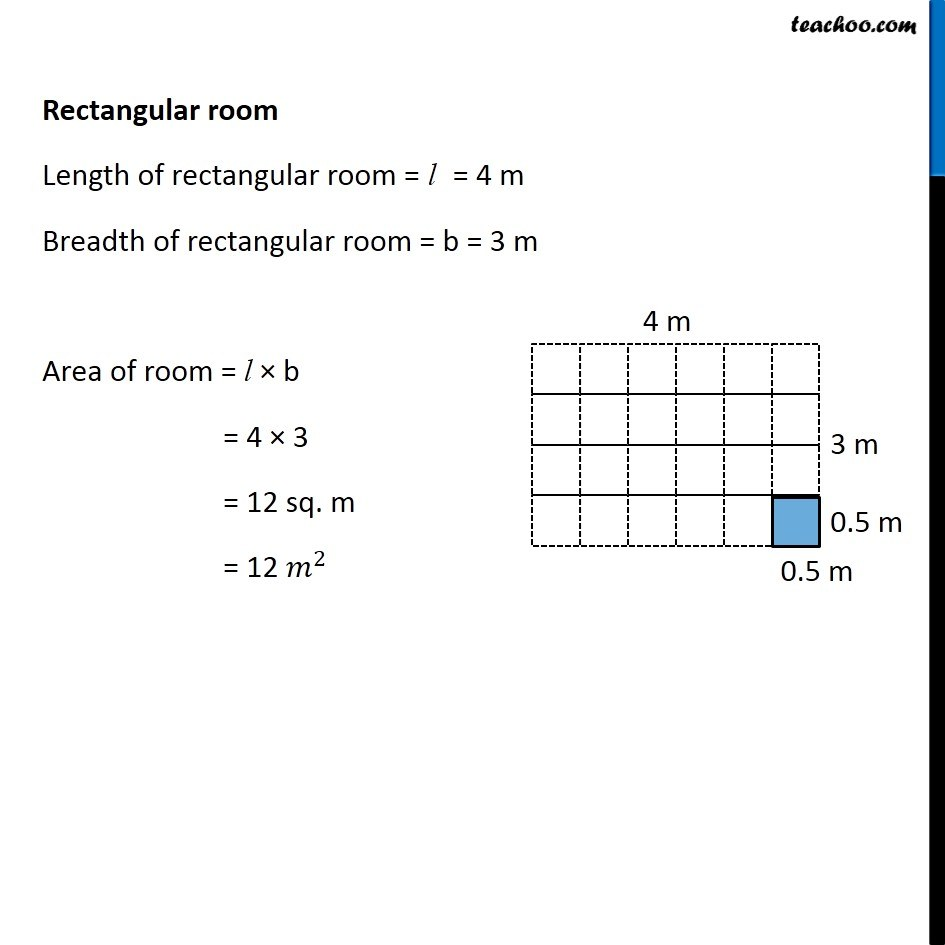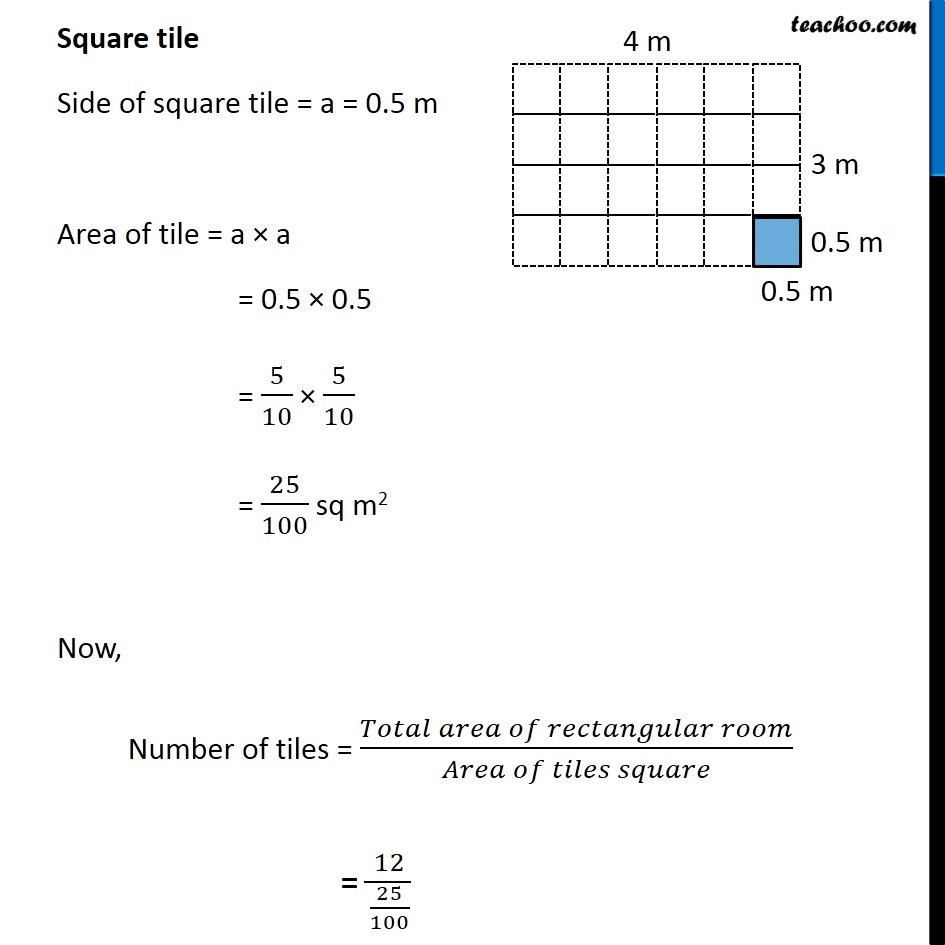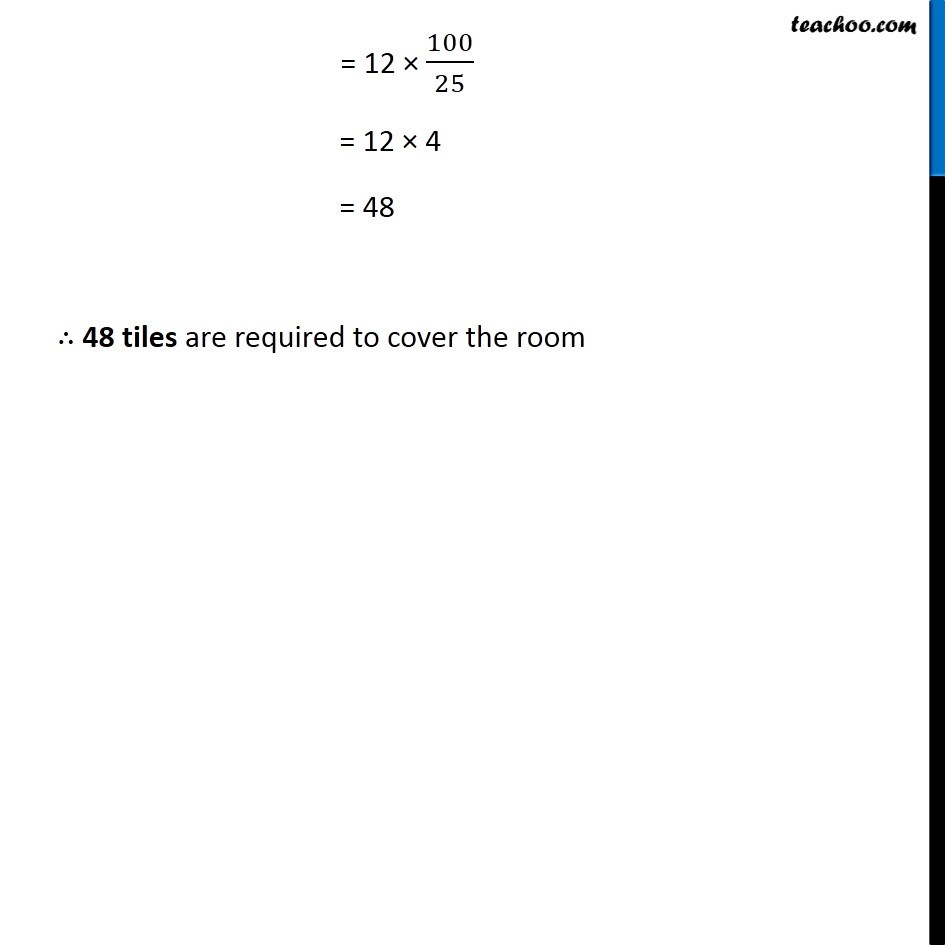Examples

Chapter 10 Class 6 Mensuration
Serial order wiseLearn in your speed, with individual attention - Teachoo Maths 1-on-1 Class

### Transcript

Example 16 Bob wants to cover the floor of a room 3 m wide and 4 m long by squared tiles. If each square tile is of side 0.5 m, then find the number of tiles required to cover the floor of the room. Here, Number of tiles = (𝑇𝑜𝑡𝑎𝑙 𝑎𝑟𝑒𝑎 𝑜𝑓 𝑟𝑒𝑐𝑡𝑎𝑛𝑔𝑢𝑙𝑎𝑟 𝑟𝑜𝑜𝑚)/(𝐴𝑟𝑒𝑎 𝑜𝑓𝑠𝑞𝑢𝑎𝑟𝑒 𝑡𝑖𝑙𝑒) Finding area of rectangular room and square tile Rectangular room Length of rectangular room = l = 4 m Breadth of rectangular room = b = 3 m Area of room = l × b = 4 × 3 = 12 sq. m = 12 𝑚^2 Square tile Side of square tile = a = 0.5 m Area of tile = a × a = 0.5 × 0.5 = 5/10 × 5/10 = 25/100 sq m2 Now, Number of tiles = (𝑇𝑜𝑡𝑎𝑙 𝑎𝑟𝑒𝑎 𝑜𝑓 𝑟𝑒𝑐𝑡𝑎𝑛𝑔𝑢𝑙𝑎𝑟 𝑟𝑜𝑜𝑚)/(𝐴𝑟𝑒𝑎 𝑜𝑓 𝑡𝑖𝑙𝑒𝑠 𝑠𝑞𝑢𝑎𝑟𝑒) = 12/(25/100) = 12 × 100/25 = 12 × 4 = 48 ∴ 48 tiles are required to cover the room